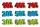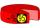3 years

The company has increased the number of employees. In year 2005 compared to 2004 13% more, in 2006 18% more against the previous year, in year 2007 20% more compared to 2006. How many % increase in the number of employees in 3 years?

Result

p =  60 %

Solution:Leave us a comment of this math problem and its solution (i.e. if it is still somewhat unclear...):Be the first to comment!To solve this verbal math problem are needed these knowledge from mathematics:

Our percentage calculator will help you quickly calculate various typical tasks with percentages.

Next similar math problems:

1. EnrollmentThe enrollment at a local college increased 4% over last year's enrollment of 8548. Find the increase in enrollment (x1) and the current enrollment (x2).
2. TVsProduction of television sets increased from 3,500 units to 4,200 units. Calculate the percentage of production increase.
3. PercentsHow many percents is 900 greater than the number 750?
4. NumberWhat number is 20 % smaller than the number 198?
5. Frameworks is badCalculate how many percent will increase the length of an HTML document, if any ASCII character unnecessarily encoded as hexadecimal HTML entity composed of six characters (ampersand, grid #, x, two hex digits and the semicolon). Ie. space as: &#x20;
6. Price increase 2xIf two consecutive times we increase the price of the product by 20%, how many % is higher final price than the original?
7. Double percentWhat is 80% of 60% of 2800?
8. Base, percents, valueBase is 344084 which is 100 %. How many percent is 384177?
9. ShotsDetermine the percentage rate of keeper interventions if from 32 shots doesn't caught four shots.
10. Sales offGoods is worth € 70 and the price of goods fell two weeks in a row by 10%. How many % decreased overall?
11. Percents - easyHow many percent is 432 out of 434?
12. Profit gainIf 5% more is gained by selling an article for Rs. 350 than by selling it for Rs. 340, the cost of the article is:
13. PercentagesExpressed as a percentage:
14. ClassIn 7.C clss are 10 girls and 20 boys. Yesterday was missing 20% of girls and 50% boys. What percentage of students missing?
15. Apples 2James has 13 apples. He has 30 percent more apples than Sam. How many apples has Sam?
16. Seeds 2How many seeds germinated from 1000 pcs, when 23% no emergence?
17. GlovesI have a box with two hundred pieces of gloves in total, split into ten parcels of twenty pieces, and I sell three parcels. What percent of the total amount I sold?